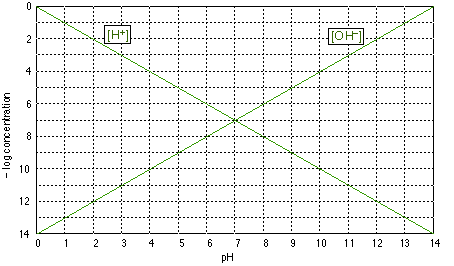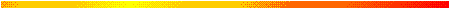### Fig. 1: Plotting the pH

Log-C vs. pH graphs are plotted on coordinates of the form shown above.Be sure you undrstand the y-axis; "4", for example, corresponds to a concentration of 10–4 M for whatever species we plot. Note that concentrations incrases with height on this graph, and that those less than about 10–8 M are usually negligible for most practical purposes.

The two plots shown on this graph are no more than formal definitions of pH and pOH; for example, when the pH is 4, –log pH = 4 and [H+] = 10–4 M. (Notice that the ordinate is the negative of the log concentration, so the smaller numbers near the top of the scale refer to larger concentrations.)

The above graph of is of no use by itself, but it forms the basis for the construction of other graphs specific to a given acid-base system. You should be able to draw this graph from memory.